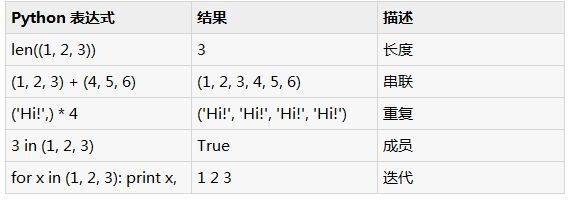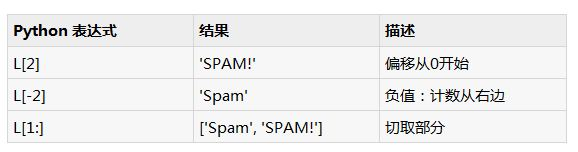# 初步讲解python中的元组概念

tup1 = (‘physics’, ‘chemistry’, 1997, 2000);
tup2 = (1, 2, 3, 4, 5 );
tup3 = “a”, “b”, “c”, “d”;

tup1 = ();

tup1 = (50,);

#!/usr/bin/python
tup1 = (‘physics’, ‘chemistry’, 1997, 2000);
tup2 = (1, 2, 3, 4, 5, 6, 7 );
print “tup1: “, tup1
print “tup2[1:5]: “, tup2[1:5]

tup1: physics
tup2[1:5]: [2, 3, 4, 5]

#!/usr/bin/python
tup1 = (12, 34.56);
tup2 = (‘abc’, ‘xyz’);
# following action is not valid for tuples
# tup1 = 100;
# so let’s create a new tuple as follows
tup3 = tup1 + tup2;
print tup3;

(12, 34.56, ‘abc’, ‘xyz’)

#!/usr/bin/python
tup = (‘physics’, ‘chemistry’, 1997, 2000, hema);
print tup;
del tup;
print “after deleting tup : ”
print tup;

(‘physics’, ‘chemistry’, 1997, 2000)
after deleting tup :
traceback (most recent call last):
file “test.py”, line 9, in
print tup;
nameerror: name ‘tup’ is not definedl = (‘spam’, ‘spam’, ‘spam!’)#!/usr/bin/python
print ‘abc’, -4.24e93, 18+6.6j, ‘xyz’;
x, y = 1, 2;
print “value of x , y : “, x,y;

abc -4.24e+93 (18+6.6j) xyz
value of x , y : 1 2

Posted in 未分类